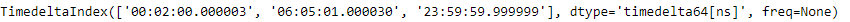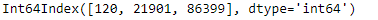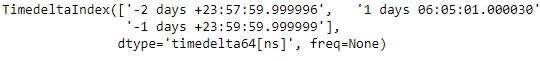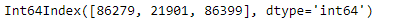Related Articles
Python | Pandas TimedeltaIndex.seconds
• Last Updated : 29 Dec, 2018

Python is a great language for doing data analysis, primarily because of the fantastic ecosystem of data-centric python packages. Pandas is one of those packages and makes importing and analyzing data much easier.

Pandas` TimedeltaIndex.seconds` attribute return the number of seconds for each element in the TimedeltaIndex object. The value returned ranges from 0 to less than 1 day.

Syntax : TimedeltaIndex.seconds

Return : index object

Example #1: Use `TimedeltaIndex.seconds` attribute to find out the seconds value for each entry of the TimedeltaIndex object.

 `# importing pandas as pd``import` `pandas as pd`` ` `# Create the TimedeltaIndex object``tidx ``=` `pd.TimedeltaIndex(data ``=``[``'2 min 3us 10ns'``, ``'06:05:01.000030'``,``                                               ``'+23:59:59.999999'``])`` ` `# Print the TimedeltaIndex``print``(tidx)`

Output :Now we will print the number of seconds in each entry of the object.

 `# find number of seconds for each element``tidx.seconds`

Output :As we can see in the output, the `TimedeltaIndex.seconds` attribute has returned the number of seconds in each element of the object.

Example #2: Use `TimedeltaIndex.seconds` attribute to find out the seconds value for each entry of the TimedeltaIndex object.

 `# importing pandas as pd``import` `pandas as pd`` ` `# Create the TimedeltaIndex object``tidx ``=` `pd.TimedeltaIndex(data ``=``[``'-1 days 2 min 3us 10ns'``, ``'1 days 06:05:01.000030'``,``                                                     ``'-1 days + 23:59:59.999999'``])`` ` `# Print the TimedeltaIndex``print``(tidx)`

Output :Now we will print the number of seconds in each entry of the object.

 `# find number of seconds for each element``tidx.seconds`

Output :As we can see in the output, the `TimedeltaIndex.seconds` attribute has returned the number of seconds in each element of the object.

Attention geek! Strengthen your foundations with the Python Programming Foundation Course and learn the basics.

To begin with, your interview preparations Enhance your Data Structures concepts with the Python DS Course.

My Personal Notes arrow_drop_up